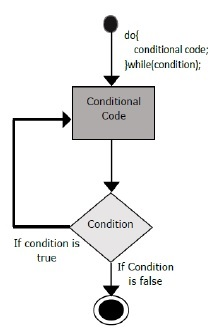# ES6 - do…while loop

The do…while loop is similar to the while loop except that the do...while loop doesn’t evaluate the condition for the first time the loop executes. However, the condition is evaluated for the subsequent iterations. In other words, the code block will be executed at least once in a do…while loop.

## FlowchartFollowing is the syntax for do-while loop in JavaScript.

```do {
Statement(s) to be executed;
} while (expression);
```

Note − Don’t miss the semicolon used at the end of the do...while loop.

## Example

```var n = 10;
do {
console.log(n);
n--;
} while(n> = 0);
```

The example prints numbers from 0 to 10 in the reverse order.

The following output is displayed on successful execution of the above code.

```10
9
8
7
6
5
4
3
2
1
0
```

### Example: while versus do…while

do…while loop

```var n = 10;
do {
console.log(n);
n--;
}
while(n> = 0);
```

while loop

```var n = 10;
while(n> = 0) {
console.log(n);
n--;
}
```

In the above example, the while loop is entered only if the expression passed to while evaluates to true. In this example, the value of n is not greater than zero, hence the expression returns false and the loop is skipped.

On the other hand, the do…while loop executes the statement once. This is because the initial iteration does not consider the boolean expression. However, for the subsequent iteration, the while checks the condition and takes the control out of the loop.